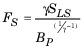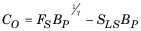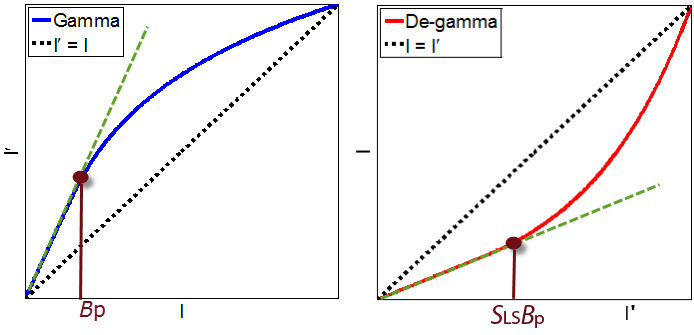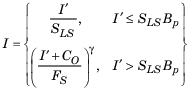Documentation

# Gamma Correction

Apply or remove gamma correction from images or video streams

## Library

Conversions

`visionconversions`## Description

Use the Gamma Correction block to apply or remove gamma correction from an image or video stream. For input signals normalized between 0 and 1, the block performs gamma correction as defined by the following equations. For integers and fixed-point data types, these equations are generalized by applying scaling and offset values specific to the data type:SLS is the slope of the straight line segment. BP is the break point of the straight line segment, which corresponds to the Break point parameter. FS is the slope matching factor, which matches the slope of the linear segment to the slope of the power function segment. CO is the segment offset, which ensures that the linear segment and the power function segments connect. Some of these parameters are illustrated by the following diagram.For normalized input signals, the block removes gamma correction, which linearizes the input video stream, as defined by the following equation:Typical gamma values range from 1 to 3. Most monitor gamma values range from 1.8 to 2.2. Check with the manufacturer of your hardware to obtain the exact gamma value. Gamma function parameters for some common standards are shown in the following table:

Standard

Slope

Break Point

Gamma

CIE L*

9.033

0.008856

3

Recommendation ITU-R BT.709-3, Parameter Values for the HDTV Standards for Production and International Programme Exchange

4.5

0.018sRGB

12.92

0.00304

2.4

### Note

This block supports intensity and color images on its ports.

The properties of the input and output ports are summarized in the following table:

PortInput/OutputSupported Data TypesComplex Values Supported

I

M-by-N matrix of intensity values or an M-by-N-by-P color video signal where P is the number of color planes

• Double-precision floating point

• Single-precision floating point

• Fixed point (up to 16-bit word length)

• 8- and 16-bit signed integer

• 8- and 16-bit unsigned integer

No

I'

M-by-N matrix of intensity values or an M-by-N-by-P color video signal where P is the number of color planes

Same as I port

No

Use the Operation parameter to specify the block's operation. If you want to perform gamma correction, select `Gamma`. If you want to linearize the input signal, select `De-gamma`.

If, for the Operation parameter, you select `Gamma`, use the Gamma parameter to enter the desired gamma value of the output video stream. This value must be greater than or equal to 1. If, for the Operation parameter, you select `De-gamma`, use the Gamma parameter to enter the gamma value of the input video stream.

Select the Linear segment check box if you want the gamma curve to have a linear portion near black. If you select this check box, the Break point parameter appears on the dialog box. Enter a scalar value that indicates the I-axis value of the end of the linear segment. The break point is shown in the first diagram of this block reference page.

## Parameters

Operation

Specify the block's operation. Your choices are `Gamma` or `De-gamma`.

Gamma

If, for the Operation parameter, you select `Gamma`, enter the desired gamma value of the output video stream. This value must be greater than or equal to 1. If, for the Operation parameter, you select `De-gamma`, enter the gamma value of the input video stream.

Linear segment

Select this check box if you want the gamma curve to have a linear portion near the origin.

Break point

Enter a scalar value that indicates the I-axis value of the end of the linear segment. This parameter is visible if you select the Linear segment check box.

## References

 Poynton, Charles. Digital Video and HDTV Algorithms and Interfaces. San Francisco, CA: Morgan Kaufman Publishers, 2003.

## See Also

 Color Space Conversion Computer Vision Toolbox™ software `imadjust` Image Processing Toolbox™ software

Download ebook